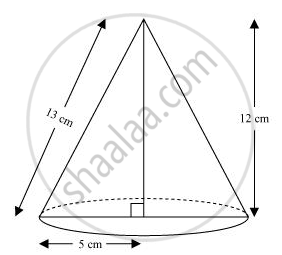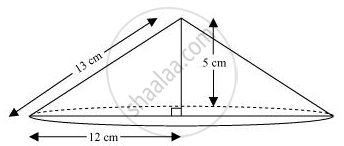# A right triangle ABC with sides 5 cm, 12 cm and 13 cm is revolved about the side 12 cm. Find the volume of the solid so obtained. - Mathematics

A right triangle ABC with sides 5 cm, 12 cm and 13 cm is revolved about the side 12 cm. Find the volume of the solid so obtained.

If the triangle ABC in the above Question is revolved about the side 5 cm, then find the volume of the solid so obtained. Find also the ratio of the volumes of the two solids obtained.

#### Solution

(i)When right-angled ΔABC is revolved about its side 12 cm, a cone with height (h) as 12 cm, radius (r) as 5 cm, and slant height (l) 13 cm will be formed.

"Volume of cone "=1/3πr^2h

=[1/3xx pi xx(5)^2xx12]cm^3

= 100π cm3

Therefore, the volume of the cone so formed is 100π cm3.

(ii)When right-angled ΔABC is revolved about its side 5 cm, a cone will be formed having radius (r) as 12 cm, height (h) as 5 cm, and slant height (l) as 13 cm.

"Volume of cone "=1/3pir^2h

=[1/3xx pi xx(12)^2xx5]cm^3

= 240π cm3

Therefore, the volume of the cone so formed is 240π cm3.

"Required ratio "= (100pi)/(240pi) = 5/12 = 5:12

Concept: Volume of a Right Circular Cone
Is there an error in this question or solution?
Chapter 13: Surface Area and Volumes - Exercise 13.7 [Page 233]

#### APPEARS IN

NCERT Class 9 Maths
Chapter 13 Surface Area and Volumes
Exercise 13.7 | Q 8 | Page 233

Share